# Maharashtra Board 11th Commerce Maths Solutions Chapter 2 Measures of Dispersion Ex 2.3

Balbharati Maharashtra State Board 11th Commerce Maths Solution Book Pdf Chapter 2 Measures of Dispersion Ex 2.3 Questions and Answers.

## Maharashtra State Board 11th Commerce Maths Solutions Chapter 2 Measures of Dispersion Ex 2.3

Question 1.
The mean and standard deviation of two distributions of 100 and 150 items are 50, 5, and 40, 6 respectively. Find the mean and standard deviation of all the 250 items taken together.
Solution: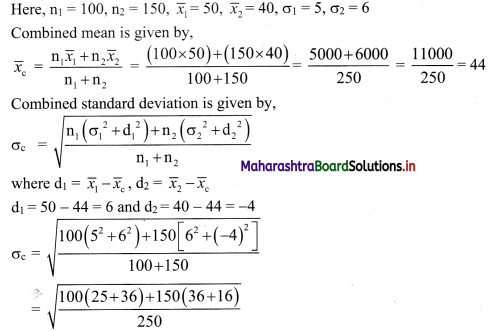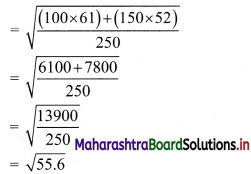∴ The mean and standard deviation of all 250 items taken together are 44 and √55.6 respectively.Question 2.
For certain bivariate data, the following information is available.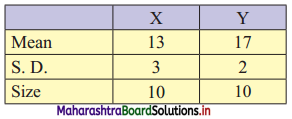Obtain the combined standard deviation.
Solution: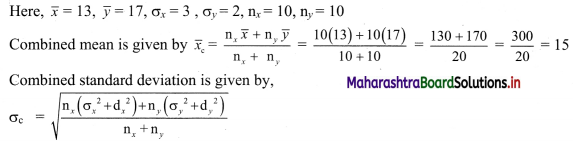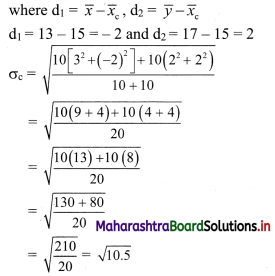Question 3.
Calculate the coefficient of variation of marks secured by a student in the exam, where the marks are: 2, 4, 6, 8, 10. (Given: √8 = 2.8284)
Solution: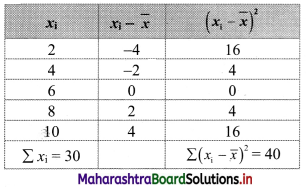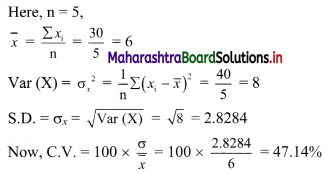Question 4.
Find the coefficient of variation of a sample that has a mean equal to 25 and a standard deviation of 5.
Solution:
Given, $$\bar{x}$$ = 25, σ = 5
C.V. = 100 × $$\frac{\sigma}{\bar{x}}$$
= 100 × $$\frac{5}{25}$$
= 20%Question 5.
A group of 65 students of class XI has their average height as 150.4 cm with a coefficient of variation of 2.5%. What is the standard deviation of their height?
Solution:
Given, n = 65, $$\bar{x}$$ = 150.4, C.V. = 2.5%
C.V. = 100 × $$\frac{\sigma}{\bar{x}}$$
∴ 2.5 = 100 × $$\frac{\sigma}{150.4}$$
∴ $$\frac{2.5 \times 150.4}{100}$$ = σ
∴ σ = 3.76
∴ the standard deviation of students’ height is 3.76.

Question 6.
Two workers on the same job show1 the following results: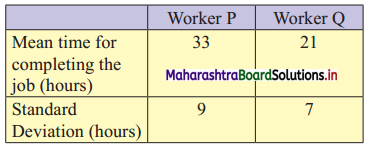(i) Regarding the time required to complete the job, which worker is more consistent?
(ii) Which worker seems to be faster in completing the job?
Solution: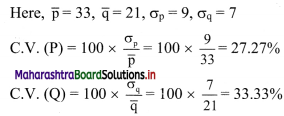(i) Since, C.V. (P) < C.V.(Q)
∴ Worker P is more consistent regarding the time required to complete the job.

(ii) Since, $$\bar{p}$$ > $$\bar{q}$$
i.e., the expected time for completing the job is less for worker Q.
∴ Worker Q seems to be faster in completing the job.Question 7.
A company has two departments with 42 and 60 employees respectively. Their average weekly wages are ₹ 750 and ₹ 400. The standard deviations are 8 and 10 respectively.
(i) Which department has a larger bill?
(ii) Which department has larger variability in wages?
Solution: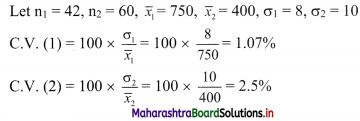(i) Since, $$\bar{x}_{1}>\bar{x}_{2}$$
i.e., average weekly wages are more for the first department.
∴ the first department has a larger bill.
(ii) Since, C.V. (1) < C.V. (2)
∴ the second department is less consistent.
∴ the second department has larger variability in wages.

Question 8.
The following table gives the weights of the students of class A. Calculate the coefficient of variation (Given √8 = 0.8944)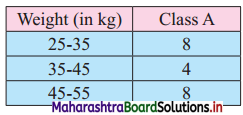Solution: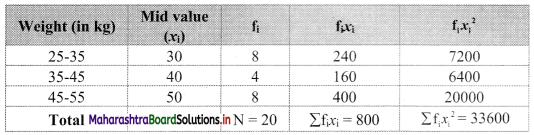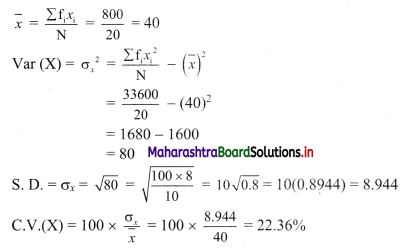Question 9.
Compute coefficient of variation for team A and team B. (Given: √2.5162 = 1.5863, √2.244 = 1.4980)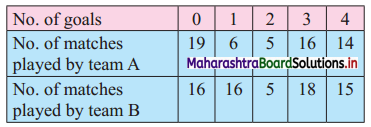Which team is more consistent?
Solution:
Let f1 denote no. of goals of team A and f2 denote no. of goals of team B.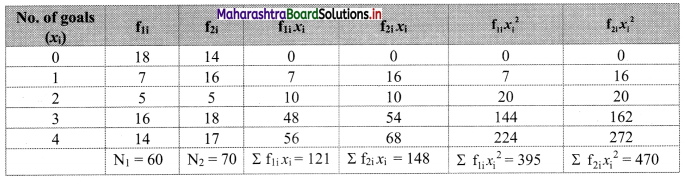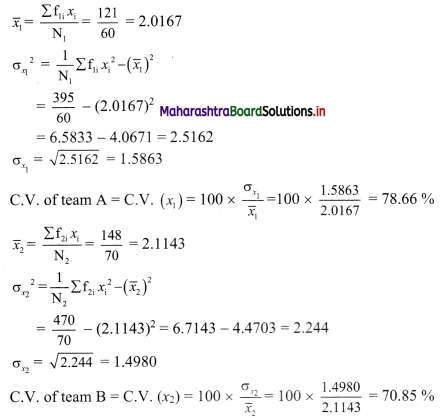Since C.V. of team A > C.V. of team B.
∴ team B is more consistent.Question 10.
Given below is the information about marks obtained in Mathematics and Statistics by 100 students in a class. Which subject shows the highest variability in marks?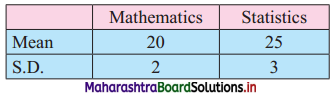Solution: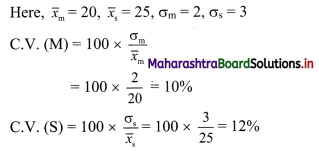Since C.V. (S) > C.V. (M)
∴ The subject statistics show higher variability in marks.